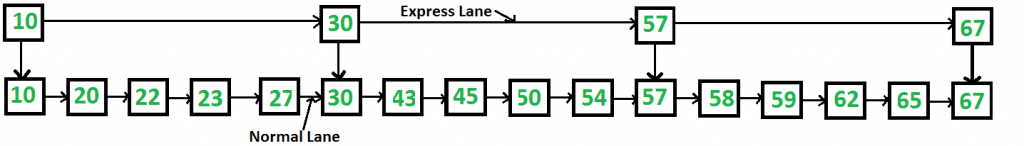# Skip List | Set 1 (Introduction)

• Difficulty Level : Easy
• Last Updated : 21 Jun, 2022

Can we search in a sorted linked list better than O(n) time? The worst-case search time for a sorted linked list is O(n) as we can only linearly traverse the list and cannot skip nodes while searching. For a Balanced Binary Search Tree, we skip almost half of the nodes after one comparison with the root. For a sorted array, we have random access and we can apply Binary Search on arrays. Can we augment sorted linked lists to search faster? The answer is Skip List. The idea is simple, we create multiple layers so that we can skip some nodes. See the following example list with 16 nodes and two layers. The upper layer works as an “express lane” that connects only the main outer stations, and the lower layer works as a “normal lane” that connects every station. Suppose we want to search for 50, we start from the first node of the “express lane” and keep moving on the “express lane” till we find a node whose next is greater than 50. Once we find such a node (30 is the node in the following example) on “express lane”, we move to “normal lane” using a pointer from this node, and linearly search for 50 on “normal lane”. In the following example, we start from 30 on the “normal lane” and with linear search, we find 50.What is the time complexity with two layers? worst-case case time complexity is several nodes on the “express lane” plus several nodes in a segment (A segment is several “normal lane” nodes between two “express lane” nodes) of the “normal lane”. So if we have n nodes on “normal lane”, √n (square root of n) nodes on “express lane” and we equally divide the “normal lane”, then there will be √n nodes in every segment of “normal lane”. √n is an optimal division with two layers. With this arrangement, the number of nodes traversed for a search will be O(√n). Therefore, with O(√n) extra space, we can reduce the time complexity to O(√n).• The skip list is solid and trustworthy.
• To add a new node to it, it will be inserted extremely quickly.
• Easy to implement compared to the hash table and binary search tree
• The number of nodes in the skip list increases, and the possibility of the worst-case decreases
• Requires only Θ(logn) time in the average case for all operations.
• Finding a node in the list is relatively straightforward.